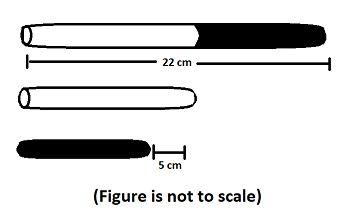QUESTION

# The diagram shown below shows two sticks- one black and the other white. Based on the measurements shown, what is the length of the white sticks?Hint The total length of the two sticks (Black and White) is 22cm, also, white is 5cm longer in length than the length of black stick. So, we get the problem minimized to solve the following equation $\left( x \right)+\left( x+5 \right)=22$. By solving this equation, we get the measurement of the both sticks.Let us assume that the length of black stick is x, then we know that the white stick is 5cm greater than the length of black stick, so the length of white stick can be written as $\left( x+5 \right)$ cm. Now, we know that the sum of both black and white sticks is 22cm. So, we get $\left( x \right)+\left( x+5 \right)=22$cm. Solving and combining the similar terms we get, $2x+5=22$cm
= $2x=22-5$
= $2x=17$, Therefore, we get the value of x as
= $x=\dfrac{17}{2}=8.5cm$. So, the length of black stick is 8.5cm and the length of white stick is $\left( 8.5+5 \right)cm$, which is 13.5cm, therefore option (c) 13.5cm is the correct answer.
Note: We can assume the length of white stick as x then the length of black stick will be $\left( x-5 \right)$ cm and our equation will become $\left( x \right)+\left( x-5 \right)=22cm$. We can solve this equation in similar steps to get the length of white stick as 13.5cm.